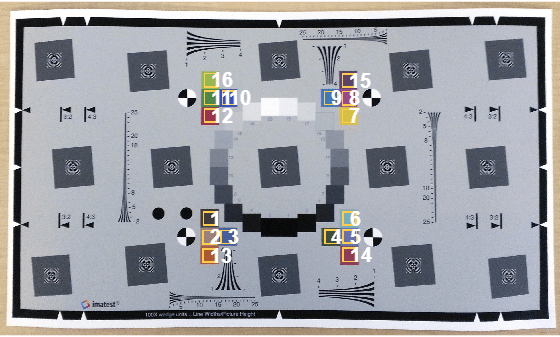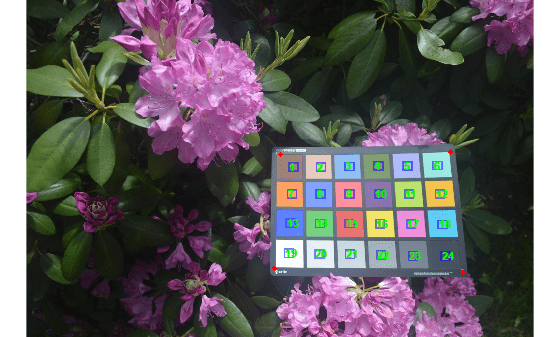# measureColor

Measure color reproduction using test chart

## Syntax

``colorTable = measureColor(chart)``
``[colorTable,colorCorrectionMatrix] = measureColor(chart)``

## Description

example

````colorTable = measureColor(chart)` measures the color values at all color regions of interest (ROIs) of an Imatest® eSFR chart  or a Calibrite ColorChecker® Classic chart .```
````[colorTable,colorCorrectionMatrix] = measureColor(chart)` also returns a color correction matrix computed using a linear least squares fit.```

## Examples

collapse all

Read an image of an eSFR chart into the workspace.

`I = imread('eSFRTestImage.jpg');`

Create an `esfrChart` object, then display the chart with ROI annotations. The 16 color patch ROIs are labeled with white numbers.

```chart = esfrChart(I); displayChart(chart,'displayEdgeROIs',false, ... 'displayGrayROIs',false,'displayRegistrationPoints',false)```Measure the color in all color patch ROIs.

`colorTable = measureColor(chart)`
```colorTable=16×8 table ROI Measured_R Measured_G Measured_B Reference_L Reference_a Reference_b Delta_E ___ __________ __________ __________ ___________ ___________ ___________ _______ 1 67 57 58 38.586 7.541 7.0812 15.15 2 156 127 122 62.182 13.225 13.826 9.8745 3 73 95 152 49.369 -0.51463 -20.062 18.455 4 62 79 58 43.926 -6.8587 17.278 14.849 5 104 109 171 53.415 9.457 -22.822 12.99 6 118 175 187 69.95 -20.889 -0.21752 13.123 7 214 192 69 78.643 1.8052 67.091 9.2399 8 154 73 138 46.853 41.998 -17.056 5.1282 9 62 120 182 51.05 -15.166 -22.416 22.813 10 55 80 185 40.811 8.7346 -44.265 22.782 11 79 135 72 55.716 -23.419 28.839 9.4826 12 152 53 77 42.759 44.167 7.9536 5.4168 13 169 91 52 58.211 27.58 47.578 15.988 14 142 63 87 47.012 39.15 8.5453 11.651 15 91 67 102 40.591 17.951 -9.525 10.897 16 152 183 80 70.505 -16.318 49.811 10.253 ```

Display the color accuracy measurements. Each square color patch is the measured color, and the thick surrounding border is the reference color for that ROI. Each color accuracy measurement is displayed as `Delta_E`, the Euclidean distance between measured and reference colors in the CIE 1976 L*a*b* color space. More accurate colors have a smaller `Delta_E`.

```figure displayColorPatch(colorTable)```For an alternative representation of the color accuracy measurements, plot the measured and reference colors in the CIE 1976 L*a*b* color space on a chromaticity diagram. Red circles indicate the reference color. Green circles indicate the measured color of each color patch. The chromaticity diagram does not portray the brightness of color.

```figure plotChromaticity(colorTable)```ROIs with a shorter distance between the reference and measurement points have smaller differences in chromaticity, which can contribute to a smaller value of `Delta_E`. However, brightness also contributes to the value of `Delta_E`. For example, even though the reference and measurement points for ROI 13 are near each other on the chromaticity diagram, they have a large `Delta_E` because of their large difference in brightness.

Read an image of a ColorChecker® chart into the workspace.

`I = imread("colorCheckerTestImage.jpg");`

Create a `colorChecker` object, then display the chart with ROI annotations.

```chart = colorChecker(I); displayChart(chart)```Measure the color in each color patch ROI.

`colorTable = measureColor(chart)`
```colorTable=24×9 table ROI Color Measured_R Measured_G Measured_B Reference_L Reference_a Reference_b Delta_E ___ ________________ __________ __________ __________ ___________ ___________ ___________ _______ 1 {'DarkSkin' } 160 129 120 37.54 14.37 14.92 20.193 2 {'LightSkin' } 229 200 191 64.66 19.27 17.5 22.587 3 {'BlueSky' } 146 191 241 49.32 -3.82 -22.54 27.312 4 {'Foliage' } 130 161 117 43.46 -12.74 22.72 20.404 5 {'BlueFlower' } 175 187 248 54.94 9.61 -24.79 23.073 6 {'BluishGreen' } 155 232 226 70.48 -32.26 -0.37 18.284 7 {'Orange' } 255 161 99 62.73 35.83 56.5 16.114 8 {'PurplishBlue'} 130 164 254 39.43 10.75 -45.17 28.889 9 {'ModerateRed' } 252 146 160 50.57 48.64 16.67 23.601 10 {'Purple' } 139 118 175 30.1 22.54 -20.87 24.672 11 {'YellowGreen' } 187 226 110 71.77 -24.13 58.19 15.21 12 {'OrangeYellow'} 241 194 76 71.51 18.24 67.37 14.148 13 {'Blue' } 96 131 255 28.37 15.42 -49.8 33.34 14 {'Green' } 118 209 130 54.38 -39.72 32.27 22.461 15 {'Red' } 234 116 114 42.43 51.05 28.62 21.87 16 {'Yellow' } 241 227 105 81.8 2.67 80.41 23.495 ⋮ ```

## Input Arguments

collapse all

Test chart, specified as an `esfrChart` object or a `colorChecker` object.

## Output Arguments

collapse all

Color values in each color patch, returned as a p-by-8 table, where p is the number of color patches on the test chart, `chart`.

The eight columns represent these variables:

VariableDescription
`ROI`Index of the sampled ROI. The value of `ROI` is an integer in the range [1, 16]. The indices match the ROI numbers displayed by `displayChart`.
`Measured_R`

Mean value of red channel pixels in the ROI. `Measured_R` is a scalar of the same data type as `chart.Image`, which can be of type `single`, `double`, `uint8`, or `uint16`.

`Measured_G`

Mean value of green channel pixels in the ROI. `Measured_G` is a scalar of the same data type as `chart.Image`.

`Measured_B`

Mean value of blue channel pixels in the ROI. `Measured_B` is a scalar of the same data type as `chart.Image`.

`Reference_L`

Reference L* value of the ROI. `Reference_L` is a scalar of type `double`.

`Reference_a`

Reference a* value of the ROI. `Reference_a` is a scalar of type `double`.

`Reference_b`

Reference b* value of the ROI. `Reference_b` is a scalar of type `double`.

`Delta_E`

Euclidean color distance between the measured and reference color values in the L*a*b* color space, as outlined in CIE 1976. `Delta_E` is a scalar of type `double`.

Color correction coefficients, returned as a 4-by-3 matrix. `colorCorrectionMatrix` represents an affine transformation that you can use to color-correct images that are captured under similar lighting conditions as the test chart image.

Data Types: `double`

## Tips

• The `measureColor` function assumes that measured RGB values are in the sRGB color space. The `measureColor` converts the values to the L*a*b* color space to calculate the color error, `Delta_E`.

• When the `chart` is an `esfrChart` object, the white point of the reference L*a*b* values is the CIE standard illuminant D65.

• When the `chart` is a `colorChecker` object, the white point of the reference L*a*b* values is the CIE standard illuminant D50. The reference L*a*b* values are for the "After November 2014" version of the ColorChecker chart.

 Calibrite. "ColorChecker Classic". https://calibrite.com/us/product/colorchecker-classic/.